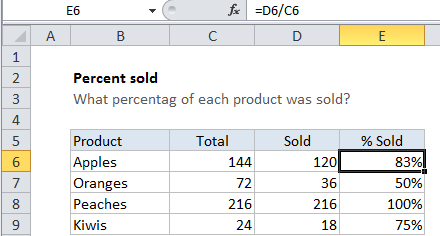## Excel Office

Excel How Tos, Tutorials, Tips & Tricks, Shortcuts

# How to calculate percent sold in Excel

To calculate percentage sold, you can use a simple formula that divides sold amount by the total amount.

## Formula

`=sold/total`Note: when working manually, percentage results are calculated by dividing one number by another, then multiplying by 100 to express as a percentage. However, because Excel can display decimal or fractional values automatically as a percentage by applying the Percentage number format, there is no need to multiply by 100.

Worked Example:   Find Percentage Change Between Numbers

## Explanation

In the example shown, the formula in E6 is

`=D6/C6`

### How this formula works

This formula simply divides the told sold by the total. In cell E6, the formula is solved like this:

```=D6/C6
=120/144
=0.83333```

When the result is formatted using the Percentage number format .83333 is displayed as 83%.